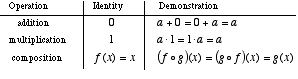index: click on a letter A B C D E F G H I J K L M N O P Q R S T U V W X Y Z A to Z index index: subject areas numbers & symbols sets, logic, proofs geometry algebra trigonometry advanced algebra & pre-calculus calculus advanced topics probability & statistics real world applications multimedia entrieswww.mathwords.com about mathwords website feedback

 Identity of an Operation The quantity which, when combined with another quantity using an operation, leaves the quantity unchanged. For example, the additive identity is 0 since x + 0 = 0 + x = x for any number x. The multiplicative identity is 1 since x·1 = 1·x = x for any number x.See also